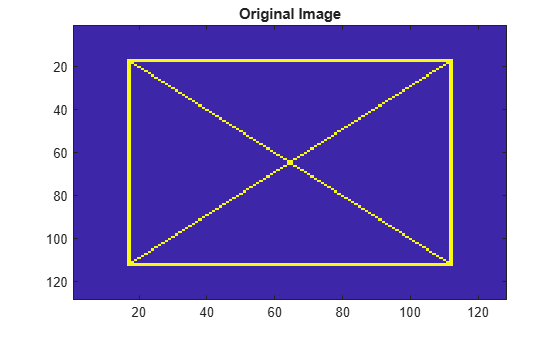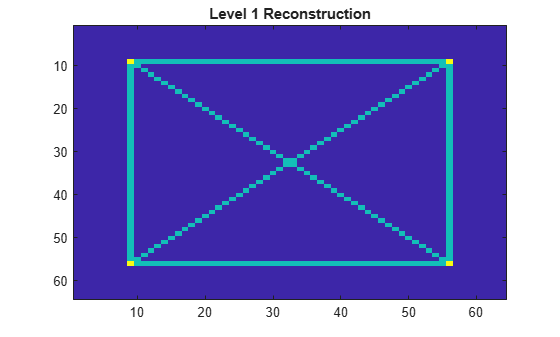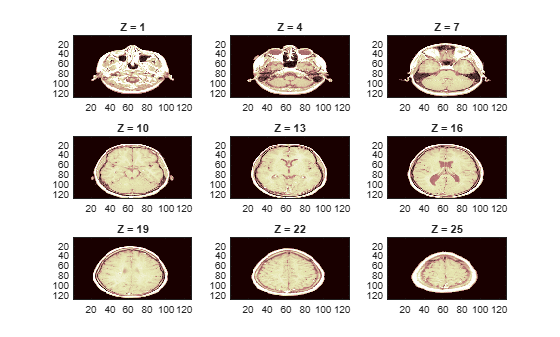# ilwt2

Inverse 2-D lifting wavelet transform

Since R2021b

## Syntax

``xr = ilwt2(ll,lh,hl,hh)``
``xr = ilwt2(ll,lh,hl,hh,Name=Value)``

## Description

example

````xr = ilwt2(ll,lh,hl,hh)` returns the 2-D inverse wavelet transform based on the approximation coefficients, `ll`, and the horizontal (`lh`), vertical (`hl`), and diagonal (`hh`) wavelet coefficients. By default, `ilwt2` assumes that you used the lifting scheme associated with the `db1` wavelet to obtain the coefficients. If you have not modified the coefficients, `xr` is a perfect reconstruction of the signal.```

example

````xr = ilwt2(ll,lh,hl,hh,Name=Value)` specifies options using one or more name-value arguments. For example, `ilwt2(ll,lh,hl,hh,LiftingScheme=lscheme,Level=3)` specifies the `lscheme` lifting scheme and the inverse transform up to level 3.```

## Examples

collapse all

Load and display the 128-by-128 `xbox` image.

```load xbox imagesc(xbox) title("Original Image")```Obtain the 2-D LWT of the image using default settings. Preserve integer values.

`[ll,lh,hl,hh] = lwt2(xbox,Int2Int=true);`

Obtain the inverse LWT up to level 1. Confirm the size of the reconstruction is 64-by-64.

```xr = ilwt2(ll,lh,hl,hh,Level=1,Int2Int=true); size(xr)```
```ans = 1×2 64 64 ```
```imagesc(xr) title("Level 1 Reconstruction")```Obtain the inverse LWT using default settings. Confirm perfect reconstruction.

```xr = ilwt2(ll,lh,hl,hh,Int2Int=true); max(abs(xr(:)-xbox(:)))```
```ans = 0 ```

Load the 3-D `wmri` data set. The data consists of 27 128-by-128 magnetic resonance images (MRI) arranged in a 128-by-128-by-27 array.

`load wmri`

Display some of the slices along the Z-orientation of the original data set.

```map = pink(90); idxImages = 1:3:size(X,3); figure("DefaultAxesXTick",[],"DefaultAxesYTick",[],... "DefaultAxesFontSize",8,"Color","w") colormap(map) for k = 1:9 j = idxImages(k); subplot(3,3,k) image(X(:,:,j)) str = sprintf("Z = %d",j); title(str) end```By default, `lwt2` performs the wavelet decomposition along the rows and columns of the input data. Use `lwt2` to obtain the 2-D LWT of each 128-by-128 slice in the 3-D data set using the lifting scheme associated with the `bior3.5` wavelet. Preserve the integer-valued data.

```lscheme = liftingScheme(Wavelet="bior3.5"); [ll,lh,hl,hh] = lwt2(X,LiftingScheme=lscheme,Int2Int=true);```

Inspect the dimensions of a detail coefficients cell array. Confirm the coefficients at each level is a 3-D array, and the size of the third dimension is 27.

`hh`
```hh=7×1 cell array {64x64x27 double} {32x32x27 double} {16x16x27 double} { 8x8x27 double} { 4x4x27 double} { 2x2x27 double} { 1x1x27 double} ```

Obtain the inverse 2-D LWT up to level 1. Confirm the size of the 3-D reconstruction is 64-by-64-by-27.

```xr = ilwt2(ll,lh,hl,hh,LiftingScheme=lscheme,Int2Int=true,Level=1); size(xr)```
```ans = 1×3 64 64 27 ```

Choose any slice from the original data set, and perform the same LWT operations on that slice. Confirm the reconstruction is equal to the corresponding slice in the 3-D reconstruction array.

```num = 13; slice = X(:,:,num); [lls,lhs,hls,hhs] = lwt2(slice,LiftingScheme=lscheme,Int2Int=true); xrs = ilwt2(lls,lhs,hls,hhs,LiftingScheme=lscheme,Int2Int=true,Level=1); max(max(abs(xrs-xr(:,:,num))))```
```ans = 0 ```

Compare the reconstruction of the slice with the original version.

```figure colormap(map) subplot(1,2,1) image(X(:,:,num)) title("Original") subplot(1,2,2) image(xrs) title("Level 1 Reconstruction")```## Input Arguments

collapse all

Approximation coefficients at the coarsest scale, specified as a scalar, vector, or matrix. The coefficients are the output of `lwt2`.

Data Types: `single` | `double`
Complex Number Support: Yes

Horizontal detail coefficients by level, specified as a LEV-by-1 cell array, where LEV is the level of the decomposition. The elements of `lh` are in order of decreasing resolution. The coefficients are the output of `lwt2`.

Data Types: `single` | `double`
Complex Number Support: Yes

Vertical detail coefficients by level, specified as a LEV-by-1 cell array, where LEV is the level of the decomposition. The elements of `hl` are in order of decreasing resolution. The coefficients are the output of `lwt2`.

Data Types: `single` | `double`
Complex Number Support: Yes

Diagonal detail coefficients by level, specified as a LEV-by-1 cell array, where LEV is the level of the decomposition. The elements of `hh` are in order of decreasing resolution. The coefficients are the output of `lwt2`.

Data Types: `single` | `double`
Complex Number Support: Yes

### Name-Value Arguments

Specify optional pairs of arguments as `Name1=Value1,...,NameN=ValueN`, where `Name` is the argument name and `Value` is the corresponding value. Name-value arguments must appear after other arguments, but the order of the pairs does not matter.

Example: ```xr = ilwt2(ll,lh,hl,hh,Wavelet="db2",Int2Int=true)```

Orthogonal or biorthogonal wavelet to use in the inverse LWT, specified as a character vector or string scalar. See the Wavelet property of `liftingScheme` for the list of supported wavelets. For perfect reconstruction, you must specify the same wavelet that you used to obtain the coefficients `ll`, `lh`, `hl`, and `hh`.

You cannot specify `Wavelet` and `LiftingScheme` at the same time.

Example: ```xr = ilwt2(ll,lh,hl,hh,Wavelet="bior3.5")``` uses the `bior3.5` biorthogonal wavelet.

Data Types: `char` | `string`

Lifting scheme to use in the inverse LWT, specified as a `liftingScheme` object. For perfect reconstruction, you must specify the same lifting scheme that you used to obtain the coefficients `ll`, `lh`, `hl`, and `hh`.

You cannot specify `LiftingScheme` and `Wavelet` at the same time.

Example: ```xr = ilwt2(ll,lh,hl,hh,LiftingScheme=lScheme)``` uses the `lScheme` lifting scheme.

Reconstruction level, specified as a nonnegative integer less than or equal to `length(hh)`-1. If you do not specify a level, the function sets the reconstruction level to `0` and `xr` is a perfect reconstruction of the signal.

Example: `xr = ilwt2(ll,lh,hl,hh,Level=2)` reconstructs the signal up to level 2.

Data Types: `double`

Extension mode to use in the inverse LWT, specified as one of these:

• `"periodic"` — Periodized extension

• `"zeropad"` — Zero extension

• `"symmetric"` — Symmetric extension

This argument specifies how to extend the signal at the boundaries.

Example: ```xr = ilwt2(ll,lh,hl,hh,Extension="zeropad")``` specifies zero extension.

Integer-valued data handling, specified as one of these:

• `1` (`true`) — Preserve integer-valued data

• `0` (`false`) — Do not preserve integer-valued data

Specify `Int2Int` only if all coefficients are integers.

Example: `xr = ilwt2(ll,lh,hl,hh,Int2Int=true)` preserves integer-valued data.

## Output Arguments

collapse all

Inverse wavelet transform, returned as a matrix. `xr` has the same dimensionality as the input used by the `lwt2` function to generate the approximation and details coefficients.

 Strang, Gilbert, and Truong Nguyen. Wavelets and Filter Banks. Rev. ed. Wellesley, Mass: Wellesley-Cambridge Press, 1997.

 Sweldens, Wim. “The Lifting Scheme: A Construction of Second Generation Wavelets.” SIAM Journal on Mathematical Analysis 29, no. 2 (March 1998): 511–46. https://doi.org/10.1137/S0036141095289051.